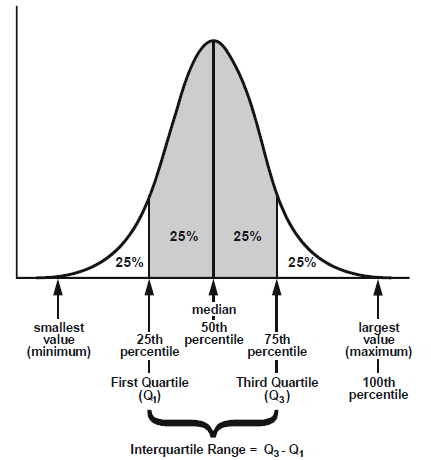# Descriptive statistics

Q. What is the meaning of Inter Quantile spread?
Q. How to plot density curve?

Hi Soumyadeep,

1. The IQR describes the middle 50% of values when ordered from lowest to highest. To find the interquartile range (IQR), ​first find the median (middle value) of the lower and upper half of the data. These values are quartile 1 (Q1) and quartile 3 (Q3). The IQR is the difference between Q3 and Q1. Here is a plot describing the same.1. Here is a link to an article explaining all about Plotting Density Curves in Python using Seaborn Library.
Histograms and Density Plots in Python

I hope it helps

Regards.